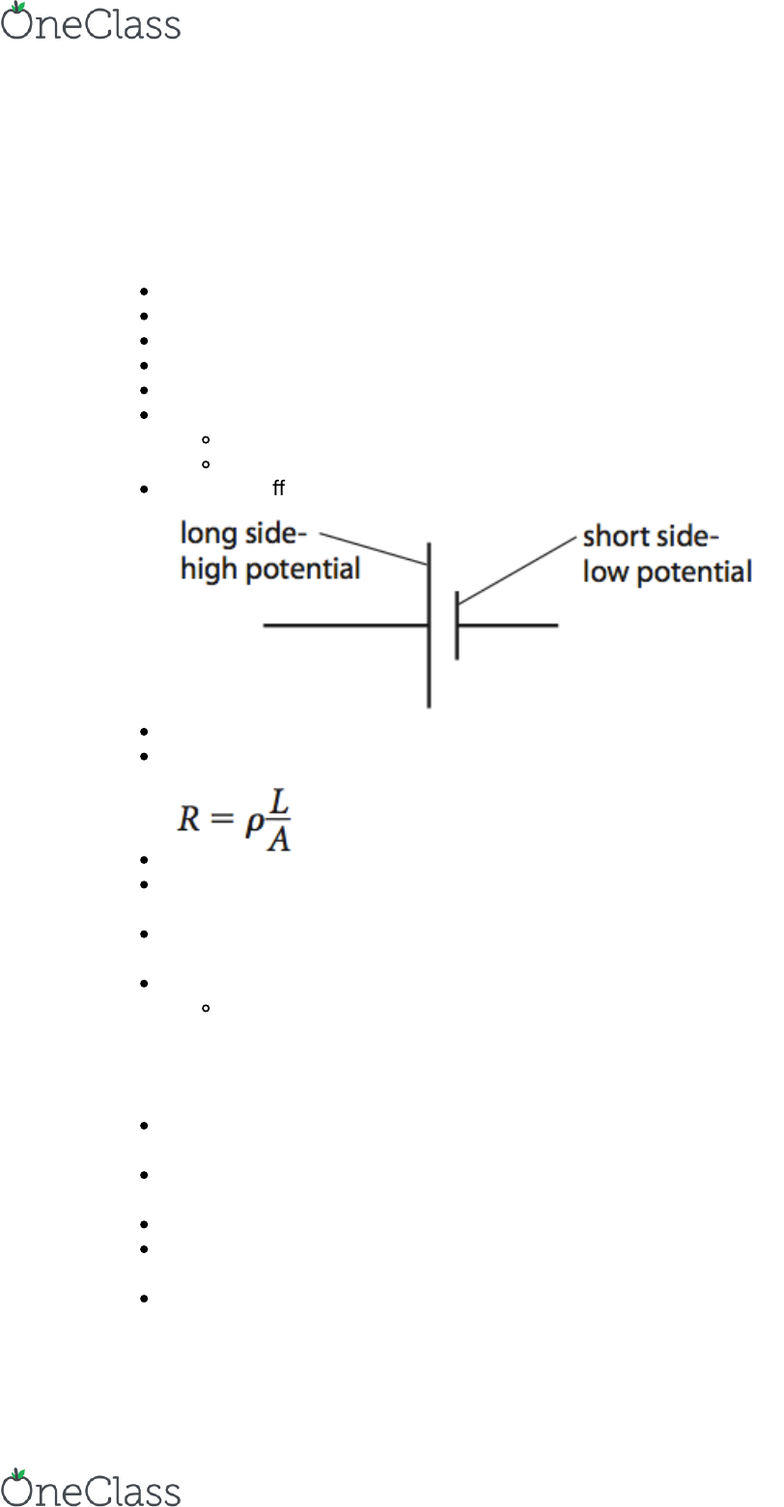Textbook Notes (270,000)
CA (160,000)
UTSG (10,000)
Physics (80)
Chapter 19

PHY152H1 Chapter Notes - Chapter 19: Series And Parallel Circuits, Internal Resistance, Electric Potential

Department
Physics
Course Code
PHY152H1
Professor
Young- June Kim
Chapter
19

Page:
of 5Electrical Currents
Electrical potential dierence, current + resistance
+ has a higher PE
Current: ﬂow of charge per unit time
current ﬂows from + —> -
Amp is a scalar quantity
Electrons are negative so they ﬂow from - to +
Battery:
chemical energy —> electrical energy
charges rearranged so that there are more + charges at the + end
Potential dierence: work done per unit charge
Resistance: rate of ﬂow of charge
ﬂow of electrons through metal give energy to metal atoms, causing temp of metal to
increase
Ohm’s law: current ﬂowing through an ohmic conductor is directly proportional to the
potential dierence across it, provided temp + physical conditions remain constant
Non-ohmic conductors e.g. light bulb
light bulb gets hot —> metal atoms vibrate more —> more collisions between
metal atoms + electrons—> increase resistance
Electric currents
emf: amount of chemical energy converted to electrical energy per unit charge (speciﬁc
to cells, batteries + generators)
Potential dierence: amount of electrical energy converted to thermal energy per unit
charge
1 volt= 1 joule per couloub
Law of conservation of energy: energy converted from chemical —> electrical in the
cell is equal to the energy converted from electrical —> heat in resistor
Internal resistance: resistance of a cell
find more resources at oneclass.com
find more resources at oneclass.com
Electrical power: rate at which energy is changed from one form to another
Power (battery): amount of chemical energy converted to electrical energy per unit
time
Series circuit:
Resistors:
same current
VT=V1+ V2
RT=R1 +R2
Cells:
VT=V1+V2
Parallel circuit:
Resistors:
same voltage
IT=I1 + I2
1/RT=1/R1 + 1/R2
Electrical measurements
voltage: voltmeter- digital + analogue
ideal voltmeter= inﬁnitely high resistance so it doesn’t take any current from the
circuit
current= ammeter
Ideal ammeter= 0 resistance so it doesn’t change the current in the circuit
Electrical sensors: a device whose electrical properties change with changing physical
conditions
Thermistor
semiconducting material
resistance decreases as temp increases
increase in temp causes the release of more charge carries —> current
can ﬂow more easily
Light sensor (LDR)
light dependent resistor
semiconducting device
light releases more charge carries resulting in lower resistance
find more resources at oneclass.com
find more resources at oneclass.com
Strain gauge
thin metal wire
stretch —>length increases —> cross-sectional area gets smaller —
> increase in resistance
Potential divider:
find more resources at oneclass.com
find more resources at oneclass.com